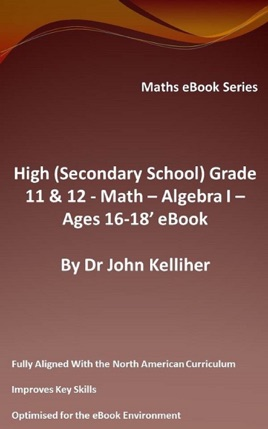• 29,00 kr

## Utgivarens beskrivning

This eBook reviews some advanced topics in algebra, including exploring the nature of polynomials, functions, equations and identity’s, examining the mathematical nomenclature used in multiplication and division. We consider multiplying out brackets, taking out common factors, manipulating algebraic fractions and simplifying expressions. Further, we include an extensive selection of questions for the student to enjoy